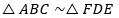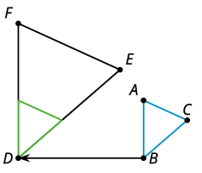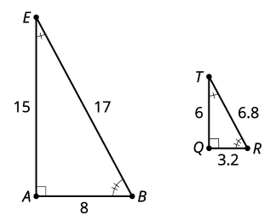# G.3.6.3 Alphabet Soup

One figure is similar to another if there is a sequence of rigid motions and dilations that takes the first figure so that it fits exactly over the second. Translation and dilation takes triangle ABC onto triangle FDE so: triangle ABC ~ triangle FDE(we read ~ as “is similar to”)Are the triangles below similar?Write a sequence of transformations (translation, rotation, reflection, dilation) to take one triangle to the other.

Write a similarity statement about the 2 figures, and explain how you know they are similar.